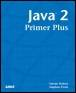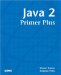# Operator PrecedenceJava™ 2 Primer PlusBy Steven Haines, Steve Potts Table of Contents Chapter 3.  Operators

There are all these operators and the good news is that they can all be used together. Consider multiplying a value by 10 and adding 5 to it:

` int result = a * 10 + 5; `

But what happens when you want to add 5 to a value, and then multiply it by 10? Does the following work?

` int result = a + 5 * 10; `

Consider for a moment that in the compiler it performs its calculations left to right, now how would you add 5 multiplied by 10 to a?

` int result = 5 * 10 + a; `

This is starting to get confusing and complicated! Furthermore it is not intuitive! When you study mathematics, you learn that certain operations are reflective, meaning that they can be performed in any order and offer the same result. Thus, the following two statements are equivalent:

` int result = a + 5 * 10;  int result = 5 * 10 + a; `

The confusion between these two statements is what operation to perform first; the multiplication or the division? How would you solve this in your math classes? You would simply instrument the statements with parentheses to eliminate the confusion:

` int result = ( a + 5 ) * 10;  int result = ( 5 * 10 ) + a; `

These statements are now read as follows: add 5 to a, and then multiply the result by 10; and multiply 5 by 10, and then add a to the result, respectively. In mathematics, the operation enclosed in parentheses is completed before any other operation.

The same mechanism can be implemented in Java, and it is! To qualify what operations to perform first, you can eliminate all ambiguity by explicitly using parentheses to denote what operations are grouped together. But that does not solve the problem of how to handle a statement without parentheses. What is the result of the following operation?

` int result = 8 + 5 * 10; `

Is the result 130 or 58?

The answer is that there needs to be a set of rules that defines the order of operation execution. This is defined by what is called the operator precedence. The operator precedence defines what operators take precedence over other operators, and hence get executed first. All programming languages define an operator precedence, which is very similar between programming languages, and you must be familiar with it.

#### Java Operator Precedence

Table 3.10 defines the operator precedence for Java. Precedence is determined by reading Table 3.10 from top to bottom, left to right.

##### Table 3.10. Operator Precedence

Type

Operators

Unary

! ~ ++ + - () new

Arithmetic

* / % + -

Shift

<< >> >>>

Comparison

< <= > >= instanceof == !=

Bitwise

& ^ |

Short-circuit

&& ||

Ternary

?:

Assignment

= "op=" (for example, *=, +=, %=, ^=)

Unary operators are those operators that operate on a single variable, such as increment and decrement (++), positive and negative signs (+ ), the bit-wise NOT operator (~), the logical NOT operator (!), parentheses, and the new operator (to be discussed later).

Arithmetic operators are those operators used in mathematical operations. Here it is important to note that this table is read from left to right, therefore multiplication and division have greater precedence than addition and subtraction. Thus the answer to the aforementioned question:

` int result = 8 + 5 * 10; `

The result is 58; multiplication has a higher precedence than addition, so the multiplication is performed first followed by the addition. So, the compiler reads this as multiply 5 by 10 (50) and add 8 to the result (50 + 8 = 58).

Shift operators refer to the bit-wise shift left, shift right, and shift right and fill with zeros operators.

The logical comparison operators follow with the familiar greater than, less than, and equality variations. Comparison operators return a boolean value, so there is one additional operator added: instanceof; this operator will be addressed later when you have a little more Java under your belt.

Next are the bitwise AND, OR, and XOR operators followed by the logical AND and OR (&& ||), referred to as the short-circuit operators.

Next is a new category of operators in the ternary operators; the sole operator in this category is referred to as ternary because it uses three operands when computing its result. It is the following form:

` a ? b : c; `

This statement is read as follows: If a is true, then the result of this operation is b, otherwise the result is c. The ternary operator does not have to be comprised of single values, the only requirement is that the first value or operation resolves to be a boolean. Consider the following examples:

` int result = ( 5 > 3 ) ? 2 : 1; // result is 2  int result = ( 5 < 3 ) ? 2 : 1; // result is 1 `

In the first example 5 is greater than 3, therefore the result is 2; in the second example 5 is not less than 3, so the result is 1. So more clearly written, the form of the ternary operator is

` (boolean expression) ? (return if true) : (return if false) `

The ternary operator is rarely used, and is mainly inherited from Java's initial syntactical base from C/C++. It is a somewhat cryptic shortcut, but is perfectly legal, so be sure to understand how it is used.

The final sets of operators in the operator precedence hierarchy are the assignment operators. The assignment operators include the familiar assignment (=) operator as well as a set of additional assignment operators referred to generically as op= (operator equal). These new operators are shortcut operators used when performing an operation on a variable and assigning the result back to that variable. Consider adding 5 to the variable a; this could be accomplished traditionally as follows:

` a = a + 5; `

Because this is such a common operation Java provides a shortcut for it:

` a += 5; `

This is read: a plus equal 5, or explicitly a equals a plus 5. The operator equal operator can be applied to all the arithmetic, shift, and bit-wise operators.

Finally, whenever there is ambiguity or you desire a higher degree of readability, you can use parentheses to explicitly qualify the operator precedence yourself.

 TopJava 2 Primer Plus
ISBN: 0672324156
EAN: 2147483647
Year: 2001
Pages: 332

Similar book on Amazon